×
Get Full Access to USC - BIOL 205 - Study Guide - Midterm
Get Full Access to USC - BIOL 205 - Study Guide - Midterm

×

USC / Biology / BIOL 205 / What is the location of most hip fractures?

# What is the location of most hip fractures? Description

##### Description: This is a summary of what will be on the next exam. Covers types of tests for statistical analysis, regression, and more. Good Luck guys :)
7 Pages 38 Views 1 Unlocks
Reviews

STATS Study Guide

## What is the location of most hip fractures?Types of Tests in this Section

Chi squared

Used tor  determine whether observed sample frequencies differ from expected  frequencies. For the chi-square test, it cannot have very small sample sizes, aka less than  5 in any cell (if it does, then use the Fisher Test).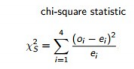Ho : p1 = p2. There is no difference between groups.

Ha : p1 > p2 (Or the Ha could be the other two options depending on the problem Ha :  p1≠p2, Ha: p1 < p2)

## How do you find the probability of an event given that another event has occurred?Don’t forget to describe what p1 and p2 are… For example: Where p1 is the probability of hip fracture without hip protectors, and p2 is the probability of hip fracture with hip  protectors

p1 and p2 are conditional probabilities (the probability of an event, given that another has  already occurred).

R code:

When in a matrix table

When given data and

probabilities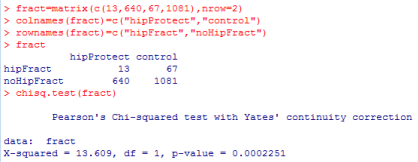type=c(111,37,34,8)

prob=c(0.5625,

0.1875, 0.1875,

0.0625)

chisq.test(type,p=

prob)

## Is number of siblings categorical or numerical?If you want to learn more check out What connects the oral cavity to the esophagus?
We also discuss several other topics like What are manipulation checks?

Df = (r - 1) * (c - 1)

where r is the number of classes for one catagorical variable, and c is the number of classes for  the other categorical variable

ex) degrees of freedom (Df) for a 2 by 2 table = 1

STATS Study Guide

Fisher Exact Test

Used when chi-square test has very small sample sizes, aka less than 5 in any cell in the  table. It gives a confidence interval for θ (odds ratio).

H0 : θ = 1

Ha: θ ≠1

Note… testing H0 : θ = 1 is the same thing as testing H0 : p1 = p2. Odds ratios are always positive and θ > 1 indicates the relative rate of success group 1 is greater than for group  2.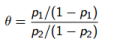If you want to learn more check out Define polygenic inheritance.

R code: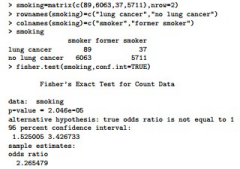The confidence interval shows how many times higher the odds of one out come are than  another. For example, if the interval is 1.232 to 3.453… the outcome increases the odds by 1.232 to 3.453 times relative to another outcome.

The odds of one outcome are (θ insert odds ratio number here) times more than the other  outcome.

STATS Study Guide

Test for Proportions

Used when Comparing probabilities of success between groups. p1 − p2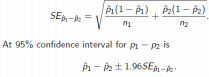Ho : p1 = p2

Ha : p1≠p2 (Or the Ha could be the other two options depending on the problem Ha : p1 > p2, Ha: p1 < p2)

R code: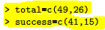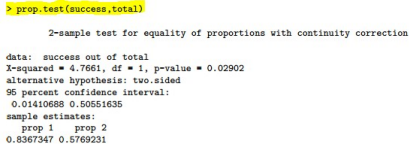To say in words use the confidence interval decimals and change them to percents We  are 95% confident that the zolendronic drug reduces hip fractures by 1.8% to 7%. We also discuss several other topics like What are the differences between classical and modernist?

Also remember: relative risk = p1/p2

STATS Study Guide

Cochran-Mantel-Haenszel test

Used when  stratified table has the same odds ratio θ1 = θ2 = · · · = θk = θs. s is for  “stratified”) is the common, conditional odds ratio ex) you have data on hospital  childbirths make a conclusion to reject the ho… the second part asks now that you have  the strata of each hospital (clinic A, clinic B, clinic C) then for the second part use this test

Simpson’s Paradox: the conclusion you make from the data changes when you break  down your data into smaller groups (When we stratify, or adjust for a third variable,  association can vanish or go in the opposite direction)

Ho : θ1 = θ2 = · · · = θk = 1

Ha : θ1 ≠ θ2 ≠ · · · ≠ θk ≠ 1

For example:Don't forget about the age old question of Can non-violence be justified without using religious grounds?

The R code is: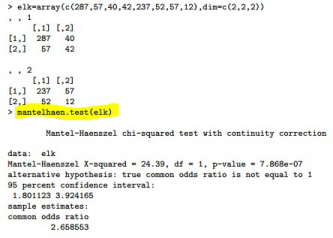STATS Study Guide

ANOVA (analysis of variance)

Used to  compare more than two means. It’s how variable the sample means y¯1,  y¯2, . . . , y¯I are to how variable observations are around each mean. It’s for continuous  variables.

Ho : µ1 = µ2 = · · · = µI

Ha : µ1 ≠ µ2 ≠ · · · ≠ µI (one or more of µ1, µ2, . . . , µI are different) If you want to learn more check out How to find the ph of a weak acid solution?

R code: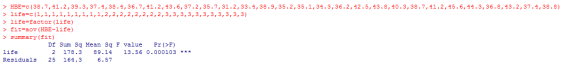(in text if you can’t see )

>HBE=c(38.7,41.2,39.3,37.4,38.4,36.7,41.2,43.6,37.2,35.7,31.2,33.4,38.9,35.2,35.1,34.3, 36.2,42.5,43.8,40.3,38.7,41.2,45.6,44.3,36.8,43.2,37.4,38.8)

> life=c(1,1,1,1,1,1,1,1,1,2,2,2,2,2,2,2,2,3,3,3,3,3,3,3,3,3,3,3)

> life=factor(life)

> fit=aov(HBE~life)

> summary(fit)

Other ANOVA Info:

Assumptions: Observations in each group are independently normally distributed with the  same variance.

Df = # of groups - 1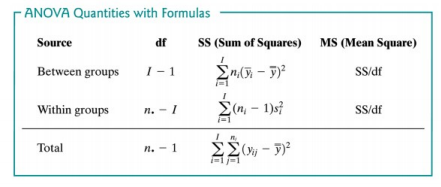The ANOVA table contains:

A MEAN SQUARE (MS): the average of the squared deviations from a central value.  It is a Sum of Squares (SS) divided by the number of informative values in the SS.  called “degrees of freedom”, or df

STATS Study Guide

Test statistic: F = MS(Between)/MS(Within)

I = number of groups

n. = number of all observations

Types of studies

Prospective studies start with a sample and observe them through time. For example,  clinical trials randomly allocate “smoking” and “non-smoking” treatments to experimental  units and then sees who ends up with lung cancer or not but we need to be ethical so  use… a cohort study

A cohort study follows subjects after letting them assign their own treatments (i.e.  smoking or non-smoking) and records outcomes.

Linear Regression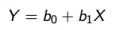Use the red circled estimate column to write out your equation from R code b0 = intercept  b1 = slope (amph in this case)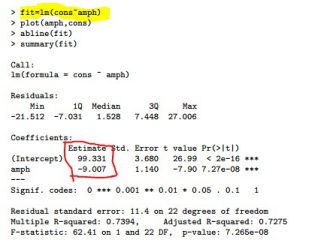Residuals: the vertical amount the points in the scatterplot missed the regression line

STATS Study Guide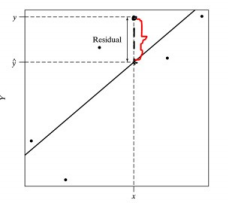Other info on regression: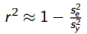is called the multiple R-squared, and is the percentage of variability in Y  explained by X through the regression line. (variation in y explained by x)

is the sample standard deviation of the Y’s. Measures the

“total variability” in the data.

is “residual standard deviation” of the Y s. Measures  variability around the regression line

Example:

> sd(weight)

 35.33766

se = 12.5 and sy = 35.3. r2 = 0.89 so 89% of the variability in weight is explained  by length.

The confidence interval for β1 = The Slope

Example:

> confint(fit)

2.5 % 97.5 %

(Intercept) 250.9778 964.4009

mass 19.3506 30.6818

…so 19.385-30.638 is for the slope

Page ExpiredIt looks like your free minutes have expired! Lucky for you we have all the content you need, just sign up here